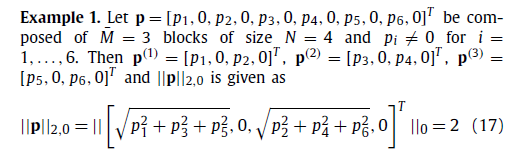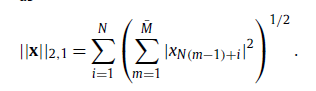# Joint Sparse (solving joint norm)

Hi
How can i solve joint norm using CVX, for solving these form of problem;

min ||X||_2,1 s.t. ||Y-HX|| < err or min ||Y-HX||_2^2 + lambda*||AX||_2,1

Regards

What exactly is the 2,1 norm?

`norms(norms(X,2,2),1,1)`
or perhaps
`norms(norms(P,1,2),2,1)`

help norms

norms Computation of multiple vector norms.
norms( X ) provides a means to compute the norms of multiple vectors
packed into a matrix or N-D array. This is useful for performing
max-of-norms or sum-of-norms calculations.

``````All of the vector norms, including the false "-inf" norm, supported
by NORM() have been implemented in the norms() command.
norms(X,P)           = sum(abs(X).^P).^(1/P)
norms(X)             = norms(X,2).
norms(X,inf)         = max(abs(X)).
norms(X,-inf)        = min(abs(X)).
If X is a vector, these computations are completely identical to
their NORM equivalents. If X is a matrix, a row vector is returned
of the norms of each column of X. If X is an N-D matrix, the norms
are computed along the first non-singleton dimension.

norms( X, [], DIM ) or norms( X, 2, DIM ) computes Euclidean norms
along the dimension DIM. norms( X, P, DIM ) computes its norms
along the dimension DIM.

Disciplined convex programming information:
norms is convex, except when P<1, so an error will result if these
non-convex "norms" are used within CVX expressions. norms is
nonmonotonic, so its input must be affine.``````
1 LikeIt looks like your example in effect reshapes a vector into a matrix, then computes the 2-norms across the rows, then sums those results.

So here is a guess, which you should check (input numerical values for X, and make sure the calculation is correct).
`sum(norms(reshape(X,M,N),2,2))`
If this isn’t correct, it should be something similar, which you should be able to figure out.

1 Like